﻿ 基于改进先验信噪比的新型单声道语音增强算法 A New Single Channel Speech Enhancement Algorithm Based on Improved A-Priori SNR

Open Journal of Circuits and Systems
Vol.07 No.03(2018), Article ID:26670,9 pages
10.12677/OJCS.2018.73010

A New Single Channel Speech Enhancement Algorithm Based on Improved A-Priori SNR

Chen Chen, Ying Gao, Shun Zhang, Ruirui Han, Shuo Zhang

School of Opto-Electronic Information, Yantai University, Yantai Shandong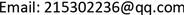Received: Aug. 11th, 2018; accepted: Aug. 24th, 2018; published: Aug. 31st, 2018ABSTRACT

Aiming at the problem of “music noise residue” existing in most speech enhancement algorithms, a new a-priori SNR estimation algorithm is proposed. Since the accuracy of the a-priori SNR estimation determines the overall performance of the speech enhancement system, the Convex-Combination (CC) algorithm is the most widely used a-priori SNR estimation algorithm. Although its real-time performance and distortion are small, its ability to suppress music noise is lacking. In order to solve this defect, this paper will improve the part of the maximum likelihood estimation in a-priori SNR estimation, and recursively smooth the a-posteriori signal-to-noise ratio by incorporating smoothing parameters, instead of the a-posteriori signal-to-noise ratio in the maximum likelihood estimation. The simulation results show that the proposed algorithm has better music noise suppression ability than CC algorithm.

Keywords:Speech Enhancement, A-Priori Signal-to-Noise Ratio, Fusion Coupling Factor, Maximum Likelihood Estimation1. 引言

DD算法由于计算简洁且容易实现，是迄今最为普及的先验信噪比估计算法。由于该算法中纯净语音谱与噪声谱相互正交的不合理假设，以及采用最大似然估计算法估计当前帧先验信噪比过程中在跟踪后验信噪比时引起一帧的时延，使得该算法音乐噪声较大。针对此缺点，后续有人提出一系列改进算法。其中运行效果较理想的是融合耦合因子算法。该算法在DD算法的基础上引入两个不同平滑参数取值的先验信噪比估计，融入一个耦合参数进行调和，在实际和估计的先验信噪比中建立代价函数求出耦合参数真实值，最终得到新的先验信噪比估计值。该算法有效避免了时延问题，能够实时跟踪信噪比的快速变化，同时失真程度大大降低。但是由于该算法在DD算法估计中采用最大似然法对后验信噪比估计，以此代替当前帧的先验信噪比估计。这使得在无语音活动区产生较大波动，输出的语音信号残留孤立峰值居多，继而产生“音乐噪声”。为了解决上述问题，本文将融合耦合因子算法进行改进，用递归平滑的方式计算后验信噪比，代替传统的瞬时后验信噪比并带入最大似然估计中，有效减少了信号的波动，同时音乐噪声抑制能力有所提升。

2. 语音增强算法的基本理论

$y\left(t\right)=x\left(t\right)+d\left(t\right)$ (1)

${Y}_{m,k}={X}_{m,k}+{D}_{m,k}$ (2)

${\stackrel{^}{X}}_{m,k}={G}_{m,k}\cdot {Y}_{m,k}$ (3)

${G}_{m,k}=\frac{{\xi }_{m,k}}{1+{\xi }_{m,k}}$ (4)

${\stackrel{^}{\xi }}_{m,k}^{DD}=\lambda {\stackrel{^}{\xi }}_{m-1,k}+\left(1-\lambda \right)\cdot {\stackrel{^}{\xi }}_{m,k}^{ML}$ (6)

${\stackrel{^}{\xi }}_{m,k}^{1}=a{\stackrel{^}{\xi }}_{m-1,k}+\left(1-a\right){\stackrel{^}{\xi }}_{m,k}^{ML}$ (7)

${\stackrel{^}{\xi }}_{m,k}^{2}=b{\stackrel{^}{\xi }}_{m-1,k}+\left(1-b\right){\stackrel{^}{\xi }}_{m,k}^{ML}$ (8)

${\stackrel{^}{\xi }}_{m,k}^{CC}=\mu {\stackrel{^}{\xi }}_{m,k}^{1}+\left(1-\mu \right){\stackrel{^}{\xi }}_{m,k}^{2}=\left(\mu a-\mu b+b\right){\stackrel{^}{\xi }}_{m-1,k}+\left(1-\mu a+\mu b-b\right){\stackrel{^}{\xi }}_{m,k}^{ML}$ (9)

$J=E\left\{{\left({\stackrel{^}{\xi }}_{m,k}^{CC}-{\xi }_{m,k}\right)}^{2}\right\}$ (10)

$\mu =\frac{\left(1-b\right){\left({\stackrel{^}{\xi }}_{m,k}^{ML}+1\right)}^{2}-b{\left({\stackrel{^}{\xi }}_{m-1,k}-{\stackrel{^}{\xi }}_{m,k}^{ML}\right)}^{2}}{\left(a-b\right)\left[{\left({\stackrel{^}{\xi }}_{m-1,k}-{\stackrel{^}{\xi }}_{m,k}^{ML}\right)}^{2}+{\left({\stackrel{^}{\xi }}_{m,k}^{ML}+1\right)}^{2}\right]}$ (11)

3. 改进先验信噪比的新型算法

${\stackrel{^}{\gamma }}_{m,k}=\beta \cdot {\stackrel{^}{\gamma }}_{m-1,k}+\left(1-\beta \right)\cdot \mathrm{min}\left[{\gamma }_{m,k},20\right]$ (12)

${\stackrel{^}{\xi }}_{m,k}^{1}=a{\stackrel{^}{\xi }}_{m-1,k}+\left(1-a\right){\stackrel{^}{\gamma }}^{ML}{}_{m,k}$ (13)

${\stackrel{^}{\xi }}_{m,k}^{2}=b{\stackrel{^}{\xi }}_{m-1,k}+\left(1-b\right){\stackrel{^}{\gamma }}^{ML}{}_{m,k}$ (14)

4. 仿真结果比较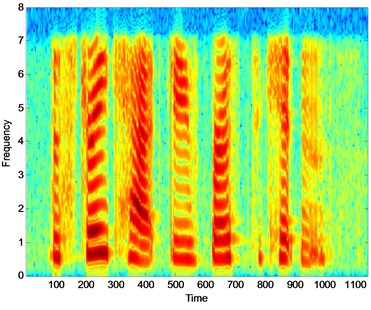(a) 纯净语音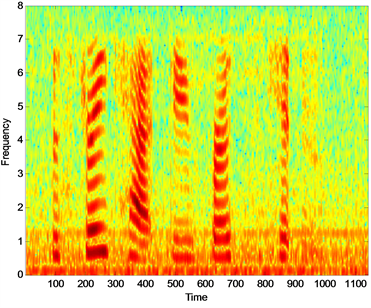(b) 带噪语音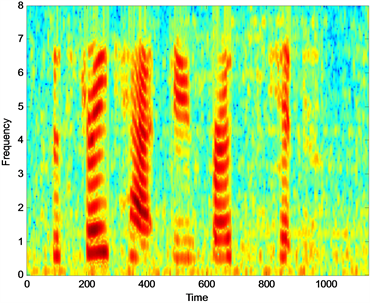(c) DD算法增强语音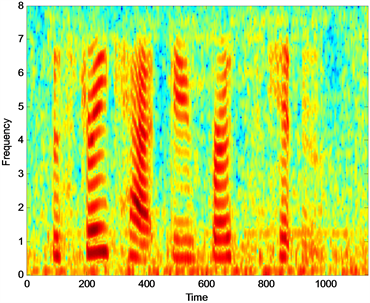(d) CC算法增强语音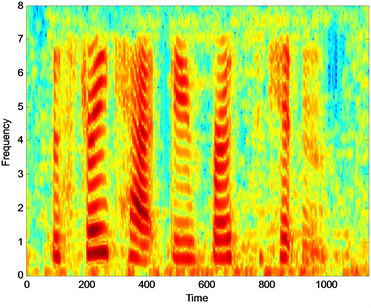(e) 改进算法增强语音

Figure 1. The spectrum of speech signal of different algorithms under M109 noise (SNR = 10 dB)Table 1. The SegSNR data comparison table of the four algorithmsTable 2. The STOI data comparison table of the four algorithms

Table 3. The LSD data comparison table of the four algorithms

5. 结论

A New Single Channel Speech Enhancement Algorithm Based on Improved A-Priori SNR[J]. 电路与系统, 2018, 07(03): 75-83. https://doi.org/10.12677/OJCS.2018.73010

1. 1. 刘伟, 陈晨, 高颖. 一种融合相位信息先验信噪比估计算法的研究[J]. 电声技术, 2017, 41(11/12): 84-87.

2. 2. Cho, J.W. and Park, H.M. (2016) Independent Vector Analysis Followed by HMM-Based Feature Enhancement for Robust Speech Recognition. Signal Process, 120, 200-208. https://doi.org/10.1016/j.sigpro.2015.09.002

3. 3. Boll, S.F. (1979) Suppression of Acoustic Noise in Speech Using Spectral Subtraction. IEEE Transactions on Acoustics, Speech, and Signal Processing, 27, 113-120. https://doi.org/10.1109/TASSP.1979.1163209

4. 4. Ephraim, Y. and Malah, D. (1984) Speech Enhancement Using a Minimum Mean-Square Error Short-Time Spectral Amplitude Estimator. IEEE Transaction on Acoustic Speech Signal Process, 32, 1109-1121. https://doi.org/10.1109/TASSP.1984.1164453

5. 5. Ephraim, Y. and Harry, L.V.T. (1995) A Signal Subspace Approach for Speech Enhancement. IEEE Transactions on Speech and Audio Processing, 3, 251-266. https://doi.org/10.1109/89.397090

6. 6. 孙海东. 基于新型先验信噪比估计的语音增强算法研究[D]: [硕士学位论文]. 烟台: 烟台大学, 2015.

7. 7. Plapous, C. and Marro, C. (2006) Improved Signal-to-Noise Ratio Estimation for Speech Enhancement. IEEE Transactions on Audio, Speech, and Language Processing, 14, 2098-2108. https://doi.org/10.1109/TASL.2006.872621

8. 8. Yong, P.C., Nordholm, S. and Dam, H.H. (2013) Optimization and Evaluation of Sigmoid Function with A Priori SNR Estimate for Real-Time Speech Enhancement. Speech Communications, 55, 358-376. https://doi.org/10.1016/j.specom.2012.09.004

9. 9. Shen, S., Ou, S., Wei, J., et al. (2017) A Priori SNR Estimator Based on a Convex Combination of Two DD Approaches for Speech Enhancement. 2016 IEEE International Conference on Signal and Image Processing, Beijing, 13-15 August 2016, 750-754.

10. 10. Hasan, T. and Hasan, Md.K. (2010) MMSE Estimator for Speech Enhance-ment Considering the Constructive and Destructive Interference of Noise. IEI Signal Processing, 4, 1-4. https://doi.org/10.1049/iet-spr.2008.0114

11. 11. 陈国明. 语音增强技术研究[D]: [博士学位论文]. 南京: 东南大学, 2007.

12. 12. 沈锁金. 语音增强技术中的先验信噪比估计算法研究[D]: [硕士学位论文]. 烟台: 烟台大学, 2017.

13. 13. Lu, Y. and Loizou, P.C. (2008) A Geometric Approach to Spectral Subtraction. Speech Communication, 50, 453. https://doi.org/10.1016/j.specom.2008.01.003

14. 14. Sun, H., Ou, S., Liu, R., et al. (2015) A Variable Momentum Factor Algo-rithm for a Priori SNR Estimation in Speech Enhancement. 2014 7th International Congress on Image and Signal Processing, Dalian, 14-16 October 2014, 888-892.

15. 15. Taal, C.H., Hendriks, R.C., Heusdens, R., et al. (2011) An Algorithm for Intelligibility Prediction of Time-Frequency Weighted Noisy Speech. IEEE Transactions on Audio Speech & Language Processing, 19, 2125-2136. https://doi.org/10.1109/TASL.2011.2114881

16. 16. Pei, C.Y., Nordholm, S. and Hai, H.D. (2013) Optimization and Evaluation of Sigmoid Function with A Priori SNR Estimate for Real-Time Speech Enhancement. Speech Communication, 55, 358-376. https://doi.org/10.1016/j.specom.2012.09.004